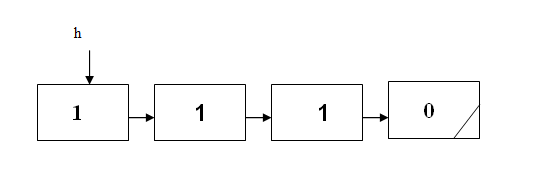# 程序设计能力实训

1215. 素进制链表

1、将素数从小到大排列成一个素数列表：$[2,3,5,7,11,13,17,19,23,29,31,\cdots]$。

2、在素数进制表示法中，从右边最低位开始，第 $n$ 位的进制定义为素数列表中第 $n$ 个素数，第 $n$ 位可用的数码为 $0$~（第 $n$ 个素数$-1$），第 $n$ 位对应的位权为素数列表中前 $n-1$ 个素数的乘积，第 $n$ 位上的数在运算时逢该素数进一位。即：右边最低位个位（第 $1$ 位）的进制为 $2$ 进制 (个位上可用的数码为 $0$，$1$)，对应的位权为 $1$；十位（第 $2$ 位）的进制为 $3$ 进制（十位上可用的数码为 $0，1，2$），对应的位权为 $2$；百位的进制为 $5$ 进制（百位上可用的数码为 $0，1，2，3，4$），对应的位权为 $23$；千位的进制为 $7$ 进制（千位上可用的数码为 $0，1，2，3，4，5，6$），对应的位权为 $23*5$，依次类推。### 样例

Input
3
2
6
38

Output
case #0:
1;0;
case #1:
1;0;0;
case #2:
1;1;1;0;


### 提示

typedef struct Node
{ char value; //存贮素进制数的一位
struct Node *next;
}NODE;